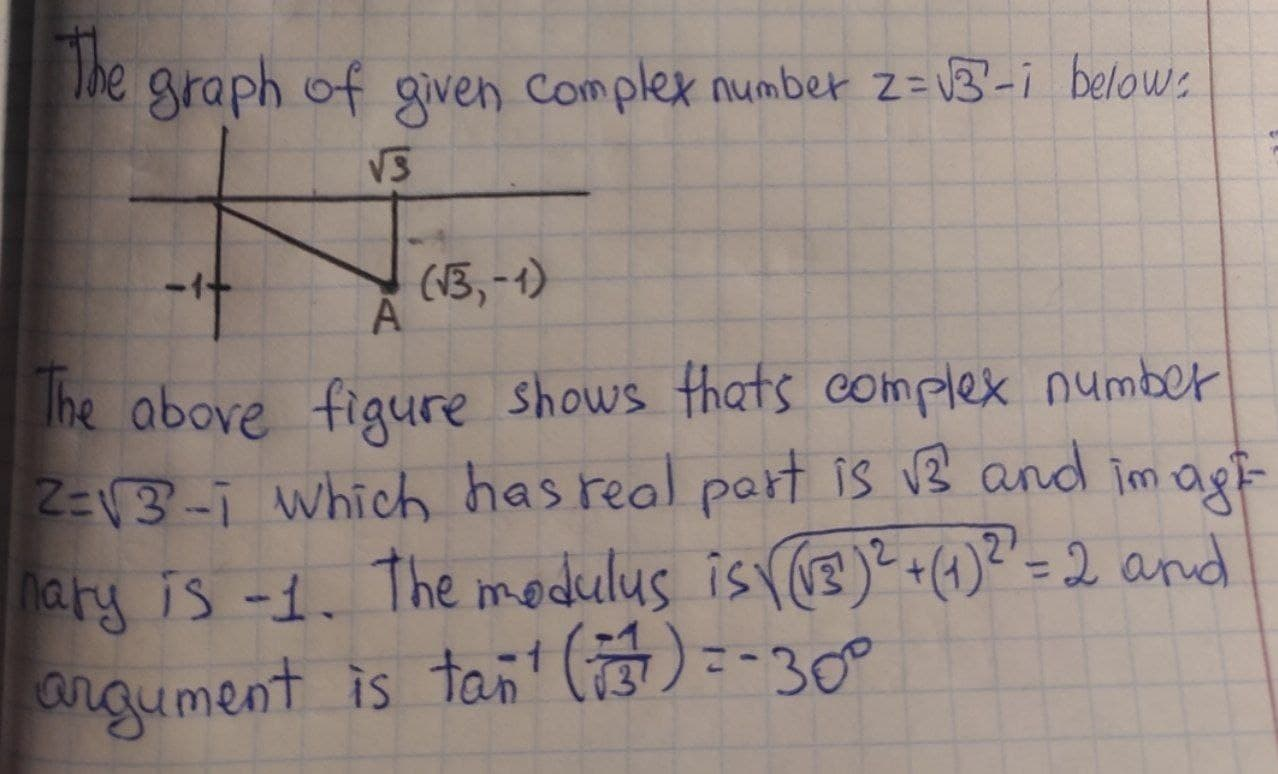# Graph the point z= \sqrt{3}-i and find the modulus andSchachtN 2021-08-10 Answered
Graph the point $z=\sqrt{3}-i$ and find the modulus and argument of z
You can still ask an expert for help

## Want to know more about Complex numbers?

• Questions are typically answered in as fast as 30 minutes

Solve your problem for the price of one coffee

• Math expert for every subject
• Pay only if we can solve itkomunidadO
To find: